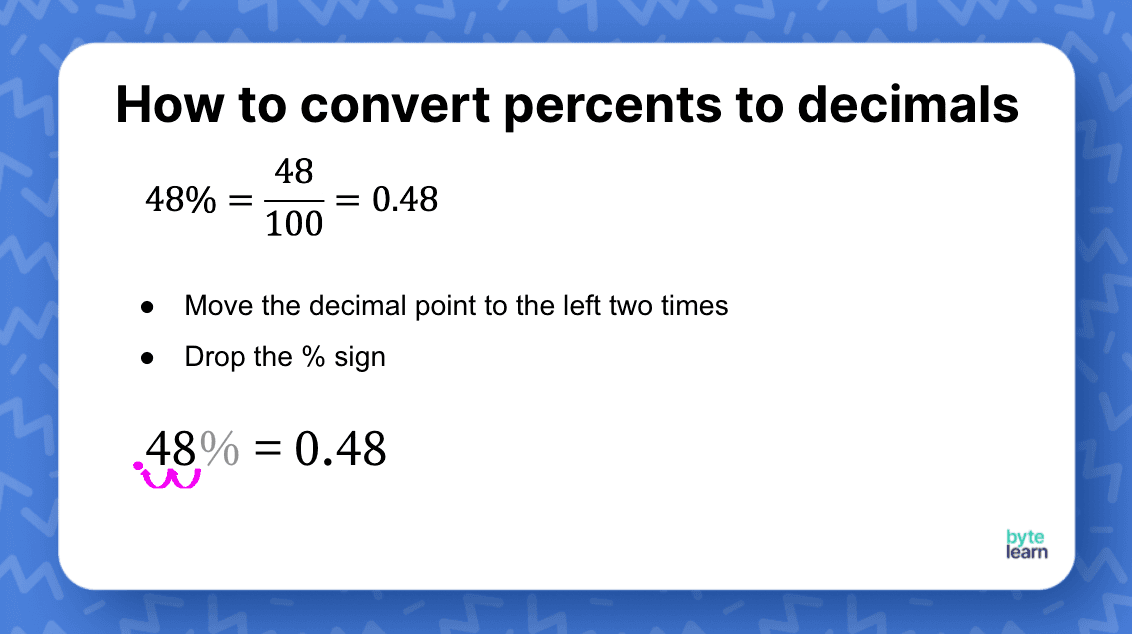ResourcesTestimonials
Plans
ResourcesTestimonials
PlansLesson plan

# Converting Between Percents and Decimals Lesson Plan - 6th Grade Math

## Overview

In this lesson, we’ll introduce converting between percents and decimals for 6th graders. Students are introduced to this lesson with a warm-up on finding the fraction, decimal, and percent of a shaded 100s grid. This prepares students to talk about percentages and decimals and how they relate to each other. Students will then work on converting percentages and decimals.

Percents
6.RP.A.3.C

## Objective

Students will be able to convert percentages into decimals and decimals into percentages.

## Materials

• Slideshow
• Anchor Chart
• Online Practice

## How to Teach Converting Between Percents and Decimals

### Warm-up

Start the lesson by having students complete a warm-up activity on finding the fraction, decimal, and percent of the 100s grid.Show students the grid where 74 boxes are filled in. Then go over the answers 74/100, 0.74, and 74% Discuss with students how the fraction, decimal, and percent all relate to each other. Share the anchor chart of Fractions, decimals, and percents and focus on “Percent to Decimal” and “Decimal to Percent.”

### Why multiply or divide by 100?

Ask students why they think we need to multiply by 100 when we convert a percent to a decimal. Eventually, we want students to understand that percent means "per 100" and that we can write a percent as a fraction over 100.

13% = 13/100 = 0.13

Similarly, when we convert a decimal to a percent, here is what happens:

0.13 = 13/100 = 13%

Over time, students will remember the process and take the shortcut, but providing this conceptual foundation will help them when they forget the process!

### Converting percents to decimals

Ask students to convert 48% to a decimal. Once students have shared their answers and how they thought about them, you can translate that into a formal process. While many students will have their way of converting percents to decimals, you also want to give the steps who would like to use it as a reference.Follow up this example with more complex problems like 7%, 7.6%, and 175%. Ask students to share their answers and how they converted. These are supposed to be quick shares. They could come up to the board to show their work. In case you want to share the answers with some students who might want to copy them down, here is a slide:

As a challenge, you could pose a question on converting 4 1/3% into a decimal. This can lead to some interesting conversations.

### Converting decimals to percents

Present a problem to students: Convert 0.36 to a percent. Students are likely to make connections to the work they just did on converting percents to decimals. Discuss their strategies and then share a slide on how to convert decimals to percents.

You can practice some more problems for converting decimals to percents as a class, or you can jump directly into independent practice. Depending on how much time you have, you could also pose more complicated problems like 0.1\overline{6}. The trick is to write a few additional digits and then move the decimal point.

At the end of the lesson, you can share an anchor chart that can act as a reference for students.

## Converting Between Percents and Decimals Practice

After you’ve completed the examples with the whole class, it’s time for some independent practice! ByteLearn gives you access to tons of converting between percents and decimals practice. Check out the online practice and assign to your students for classwork and/or homework! You should try a combination of mild, medium, and spicy problems!!Converting Between Percents and Decimals Practice